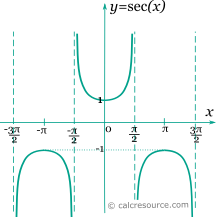Jump to
Table of Contents
Share this

## Trigonometric secant calculator

This tool evaluates the secant of a number: sec(x). Enter the argument x, in radians or degrees, below.

 x = deg rad Result: sec(x) = Also, at same x: csc(x) = cos(x) =Table of Contents
Share this

## Definitions

### General

The secant function, in modern notation written as sec(x), is a trigonometric function. Trigonometric functions are commonly established as functions of angle, in the context of right triangle geometry. This way, the secant of an angle φ is defined as the ratio of the hypotenuse divided by the adjacent side of a right triangle containing φ (see figure):The above definition of secant function assumes that its argument is an angle. This association to an angle is restrictive, considering the broad use of trigonometric functions in mathematics, physics, engineering etc. Applications may accept any real value as argument, with any imaginable meaning given to it, or no meaning at all. In that case, x should be better measured in radians (). Thus, derivation and integration rules are more conveniently applied.

### Series

All trigonometric functions can be defined in an infinite series form. Secant function can be written as:

The above series converges for . Endenotes the n-th Euler number .

### Properties

The secant function is periodic, with a period equal to 2π. Therefore:

Secant is the reciprocal of cosine:

The derivative of the secant function (x in radians) is given by the following expressions:

The integral of the secant function (x in radians) is given by: